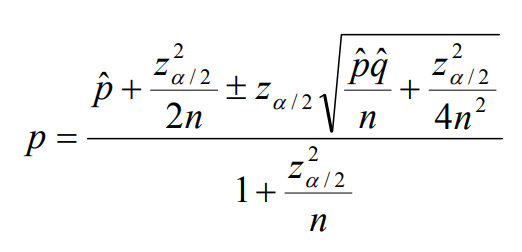# Wilson CI

Wilson CI (also called “plus-4” confidence intervals or Wilson Score Intervals) are Wald intervals computed from data formed by adding 2 successes and 2 failures. The Wilson confidence intervals  have better coverage rates for small samples. For example, you might be expecting a 95% confidence interval but only get 91%; the Wald CI can shrink this coverage issue .

For binomial confidence intervals, the Wilson CI performs much better than the normal approximation interval for small samples (e.g., n = 10) or where p is close to 0 or 1). It is preferred to the Clopper-Pearson exact method (which uses the F distribution) and the asymptotic confidence interval (the “textbook”) method [3, 4].

## Wilson CI Formula

Although the Wilson CI gives better coverage than many other methods, the algebra is more involved; the calculation involves a quadratic equation and a “complicated solution” :The math may not be an issue as many statistical software programs can calculate the Wilson CI, including R . SPSS does not have a procedure, but it is “relatively easy” to produce them with COMPUTE commands .

## References

Graph of Wilson CI: Sean Wallis via Wikimedia Commons. CC by 4.0.
 Wilson, E. B. (1927). Probable inference, the law of succession, and statistical inference. Journal of the American Statistical Association. 22 (158): 209–212. doi:10.1080/01621459.1927.10502953. JSTOR 2276774.
 Confidence intervals – Proportions – Wilson Score Interval. Retrieved February 25, 2022 from: https://www.cpp.edu/~jcwindley/classes/sta2260/Confidnece%20Intervals%20-%20Proportions%20-%20Wilson.pdf
 Confidence Intervals for Binomial Probabilities. Retrieved February 25, 2022 from: http://math.furman.edu/~dcs/courses/math47/R/library/Hmisc/html/binconf.html
 A. Agresti and B.A. Coull, Approximate is better than “exact” for interval estimation of binomial proportions, American Statistician, 52:119–126, 1998.
 Dunnigan, K. (2008). Confidence Interval Calculation for Binomial Proportions.
 RDocumentation. wilson.ci: Confidence Intervals for Proportions. Retrieved February 25, 2022 from: https://www.rdocumentation.org/packages/fastR/versions/0.10.3/topics/wilson.ci
. IBM. Can SPSS produce Wilson or score confidence intervals for a binomial proportion?

CITE THIS AS:
Stephanie Glen. "Wilson CI" From StatisticsHowTo.com: Elementary Statistics for the rest of us! https://www.statisticshowto.com/wilson-ci/